# Finite Fourier Transform Matrix

This is the third in a series of posts on the finite Fourier transform. The Fourier matrix produces an interesting graphic and has a surprising eigenvalue distribution.

### Contents

#### Fourier Matrix

The Fourier matrix of order $n$ is the $n$ -by- $n$ complex Vandermonde matrix $F$ whose elements $f_{k,j}$ are powers of the $n$ th root of unity $$\omega = e^{-2 \pi i/n}$$ $$f_{k,j} = \omega^{k j}$$ The matrix can be generated with the MATLAB statements
k = (0:n-1)';
j = (0:n-1);
F = exp(-2*pi*i*k*j/n);

Or, by taking the FFT of the identity matrix
F = fft(eye(n))

The statement
plot(F)
connects points in the complex plane whose coordinates are the real and imaginary parts of the elements of the columns of F, thereby generating a subgraph of the graph on n points. If n is prime, connecting the elements of all columns generates the complete graph on n points. If n is not prime, the sparsity of the graph of all columns is related to the computational complexity, and hence the speed, of the fast FFT algorithm. The graphs for n = 8, 9, 10, and 11 are generating and plotted by the following code.
   for n = 8:11
subplot(2,2,n-7)
F = fft(eye(n));
plot(F)
axis square
axis off
title(['n = ' int2str(n)])
end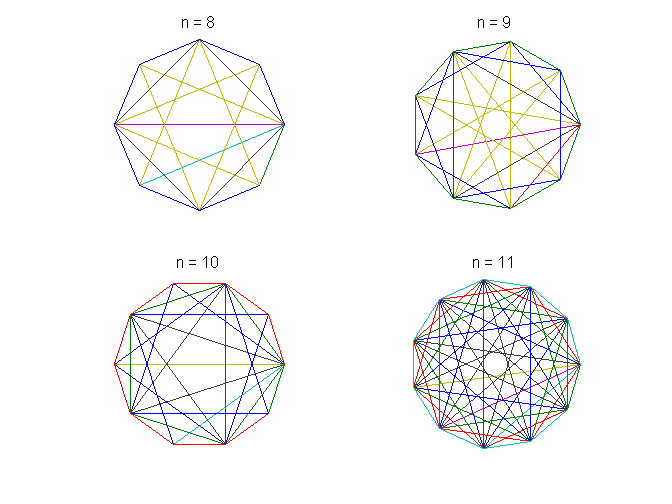Because n = 11 is prime, the corresponding graph shows all possible connections. But the other three values of $n$ are not prime. Some of the links in their graphs are missing, indicating that the FFT of a vector with that many points can be computed more quickly.

#### n = 12

The remainder of this post examines F12 in more detail. Here is its graph.
   clf
n = 12;
F = fft(eye(n));
plot(F)
axis square
axis off
title(['n = ' int2str(n)])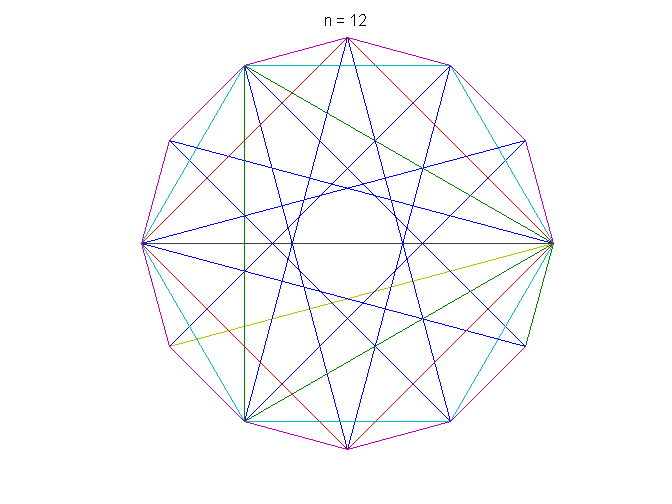#### fftmatrix

The program fftmatrix, available here, or included with the NCM App, allows you to investigate these graphs. fftmatrix(n) plots all the columns of the Fourier matrix of order n. fftmatrix(n,j) plots just one column. Let's plot the individual columns of F12. The first column of F12 is all ones, so its plot is just a single point.
   for j = 1:12
p = mod(j-1,4)+1;
subplot(2,2,p);
fftmatrix_mod(12,j-1)
title(['j = ' int2str(j)])
if p == 4, snapnow, end
end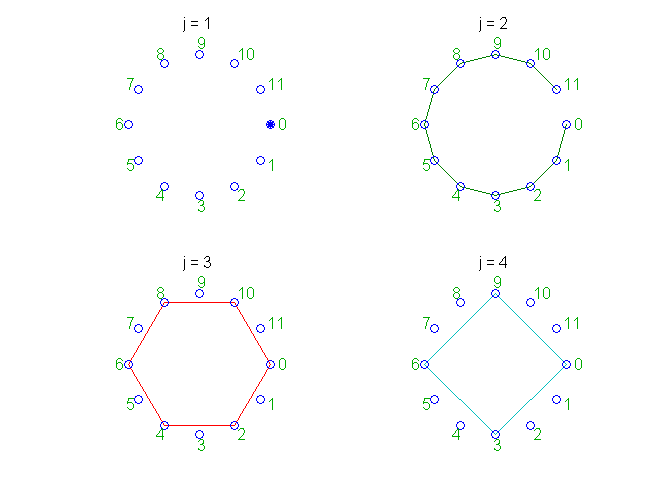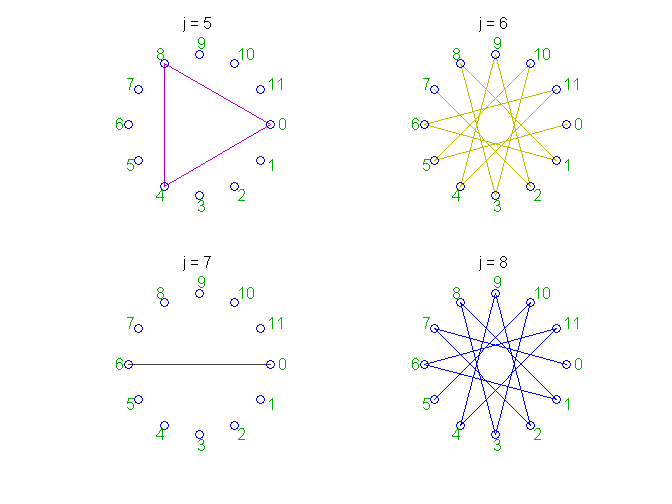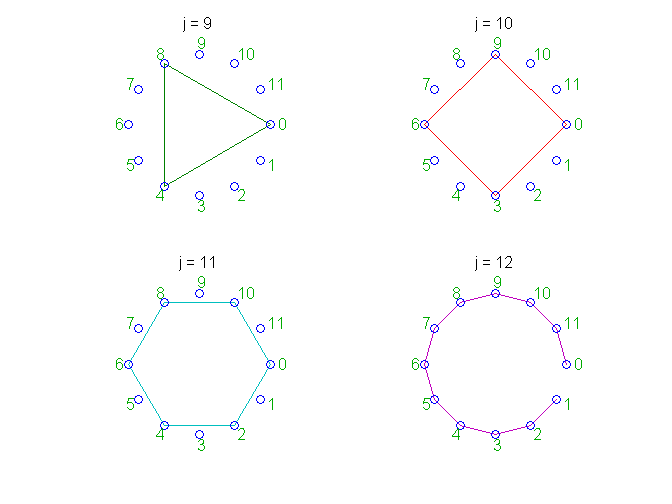To see typical behavior, look at the third subplot, the red graph labeled j = 3, generated by the third column
   F(:,3)

ans =
1.0000 + 0.0000i
0.5000 - 0.8660i
-0.5000 - 0.8660i
-1.0000 + 0.0000i
-0.5000 + 0.8660i
0.5000 + 0.8660i
1.0000 + 0.0000i
0.5000 - 0.8660i
-0.5000 - 0.8660i
-1.0000 + 0.0000i
-0.5000 + 0.8660i
0.5000 + 0.8660i

These are the powers of $\omega^2$. Because 2 divides 12 evenly these powers hit all the even-numbered points around the circle twice and miss all the odd-numbered points. Now look at the cyan graph labeled j = 11. These are the powers of $\omega^{10}$, which are the complex conjugates of the powers of $\omega^2$. So the two graphs lie on top of each other. Six of the twelve columns in the graph of F12 connect only a subset of the nodes and ten of the columns lie on top of their complex conjugate columns. As a result, when all of the columns are combined to form the full graph, it is sparse. This sparsity, in turn, makes it possible to construct a fast finite Fourier transform algorithm for n = 12.

#### Eigenvalues

I've always been curious about the eigenvalues and vectors of the Fourier matrices. In 1979, three friends of mine at the University of New Mexico, Gus Efroymson, Art Steger, and Stan Steinberg, posed the question in the SIAM Review problem section. They didn't know that Jim McClellan and Tom Parks had actually solved their problem seven years earlier, when Jim was a grad student working under Tom at Rice. I didn't learn about the McClellan-Parks paper until fairly recently. The Wikipedia page on the discrete Fourier transform says the facts about the eigenvalues are well known, but that the eigenvectors are the subject of ongoing research. Let's rescale $F$ so that its columns have unit length.
   F = F/sqrt(n);

This makes $F' \ F = I$
   round(F'*F)

ans =
1     0     0     0     0     0     0     0     0     0     0     0
0     1     0     0     0     0     0     0     0     0     0     0
0     0     1     0     0     0     0     0     0     0     0     0
0     0     0     1     0     0     0     0     0     0     0     0
0     0     0     0     1     0     0     0     0     0     0     0
0     0     0     0     0     1     0     0     0     0     0     0
0     0     0     0     0     0     1     0     0     0     0     0
0     0     0     0     0     0     0     1     0     0     0     0
0     0     0     0     0     0     0     0     1     0     0     0
0     0     0     0     0     0     0     0     0     1     0     0
0     0     0     0     0     0     0     0     0     0     1     0
0     0     0     0     0     0     0     0     0     0     0     1

Now $F$ is unitary, the complex generalization of orthogonal. This implies that all of its eigenvalues lie on the unit circle in the complex plane. Furthermore, it turns out that $F^4 = I$.
   round(F^4)

ans =
1     0     0     0     0     0     0     0     0     0     0     0
0     1     0     0     0     0     0     0     0     0     0     0
0     0     1     0     0     0     0     0     0     0     0     0
0     0     0     1     0     0     0     0     0     0     0     0
0     0     0     0     1     0     0     0     0     0     0     0
0     0     0     0     0     1     0     0     0     0     0     0
0     0     0     0     0     0     1     0     0     0     0     0
0     0     0     0     0     0     0     1     0     0     0     0
0     0     0     0     0     0     0     0     1     0     0     0
0     0     0     0     0     0     0     0     0     1     0     0
0     0     0     0     0     0     0     0     0     0     1     0
0     0     0     0     0     0     0     0     0     0     0     1

This implies that any eigenvalue $\lambda$ must satisfy $\lambda^4$ = 1. Consequently the only possible eigenvalues are 1, -1, i, and -i. You might guess that guess that if $n$ is divisible by 4, the eigenvalues would be equally distributed among these four values. But, surprisingly, to me at least, that doesn't happen.
   lambda = eig(F)

lambda =
1.0000 + 0.0000i
-1.0000 + 0.0000i
-0.0000 + 1.0000i
-1.0000 + 0.0000i
-1.0000 - 0.0000i
-0.0000 + 1.0000i
0.0000 - 1.0000i
1.0000 + 0.0000i
-0.0000 - 1.0000i
1.0000 - 0.0000i
1.0000 - 0.0000i
0.0000 - 1.0000i

It's hard to pick through this unsorted output, but there are four +1's, three -1's, three -i's, and only two +i's. Here is a tricky piece of code that uses angle and the counting feature of sparse indexing to count the number of each of the four possible eigenvalues.
   type eigfftmat

function c = eigfftmat(n)
% EIGFFTMAT Count eigenvalues of the Fourier matrix.
% c = eigfftmat(n) is a 4-vector with counts for +1, -1, -i, +i.
% Compute the eigenvalues.
e = eig(fft(eye(n)));
% Sparse repeated indexing acts as a counter.
c = full(sparse(mod(round(angle(e')/(pi/2)),4)+1,1,1))';
c([3 2]) = c([2 3]);
end


When we run this code for a sequence of values of n, we see the pattern predicted by the McClellan-Parks analysis. The number of each of the four possible eigenvalues depends upon floor(n/4) and mod(n,4).
   disp('     n    +1    -1    -i    +i')
for n = 4:20
disp([n eigfftmat(n)])
end

     n    +1    -1    -i    +i
4     2     1     1
5     2     1     1     1
6     2     2     1     1
7     2     2     2     1
8     3     2     2     1
9     3     2     2     2
10     3     3     2     2
11     3     3     3     2
12     4     3     3     2
13     4     3     3     3
14     4     4     3     3
15     4     4     4     3
16     5     4     4     3
17     5     4     4     4
18     5     5     4     4
19     5     5     5     4
20     6     5     5     4

The proofs in the McClellan and Parks paper involve the eigenvectors and are quite complicated. It turns out that this MATLAB expression
floor((n+[4 2 1 -1])/4)

generates a 4-vector of the multiplicities of the +1, -1, -i, and +i eigenvalues for any given value of n.

#### References

J. H. McClellan and T. W. Parks, "Eigenvalues and eigenvectors of the discrete Fourier transformation", IEEE Trans. Audio Electroacoust 20, 66-74, <http://dx.doi.org/10.1109/TAU.1972.1162342>, 1972. G. Efroymson, A. Steger, and S.Steinberg, "A Matrix Eigenvalue Problem", SIAM Review, 21, 139-139, <http://dx.doi.org/10.1137/1021013>, 1979. Wikipedia, "Discrete Fourier Transform", <http://en.wikipedia.org/wiki/Discrete_Fourier_transform>, retrieved 09/03/2014.

Published with MATLAB® R2014a

|